# Useful Equations and Constants AG AG RTIn Q R 8.314olk F96485% vg > .G7,reactants 22, m Zi Ink互=...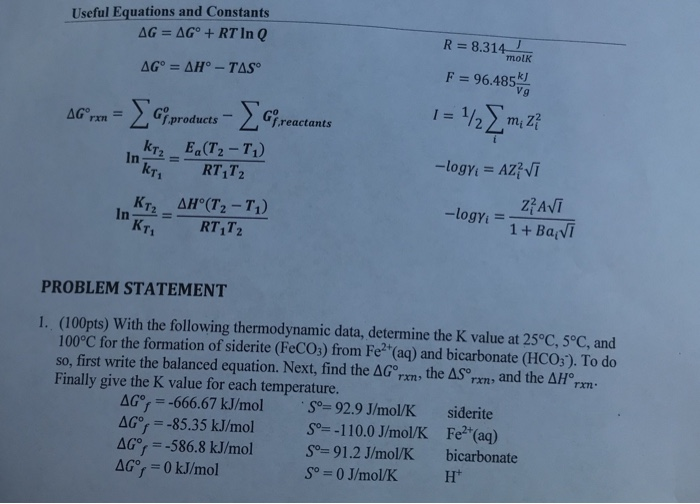Useful Equations and Constants AG AG RTIn Q R 8.314olk F96485% vg > .G7,reactants 22, m Zi Ink互= Ea(T2-T1) 112 ΔΗ'(T2-T1) In- T1 =-T1T2 PROBLEM STATEMENT (100pts) With the following thermodynamic data, determine the K value at 25°C, 5°C, and 100°C for the formation of siderite (FeCOs) from Fe2 (aq) and bicarbonate (HCO;). To do so, first write the balanced equation. Next, find the ΔGorm, the ASorm, and the ΔΗ0rm. Finally give the K value for each temperature. 1. ΔG。--666.67 kJ/mol . So-929 J/mol/K siderite AG 85.35 kJ/mol110.0 J/mol/K dGor =-586.8 kJ/mol Soー91.2 J/mol/K Fe (aq) bicarbonate Ht So 0 J/mol/K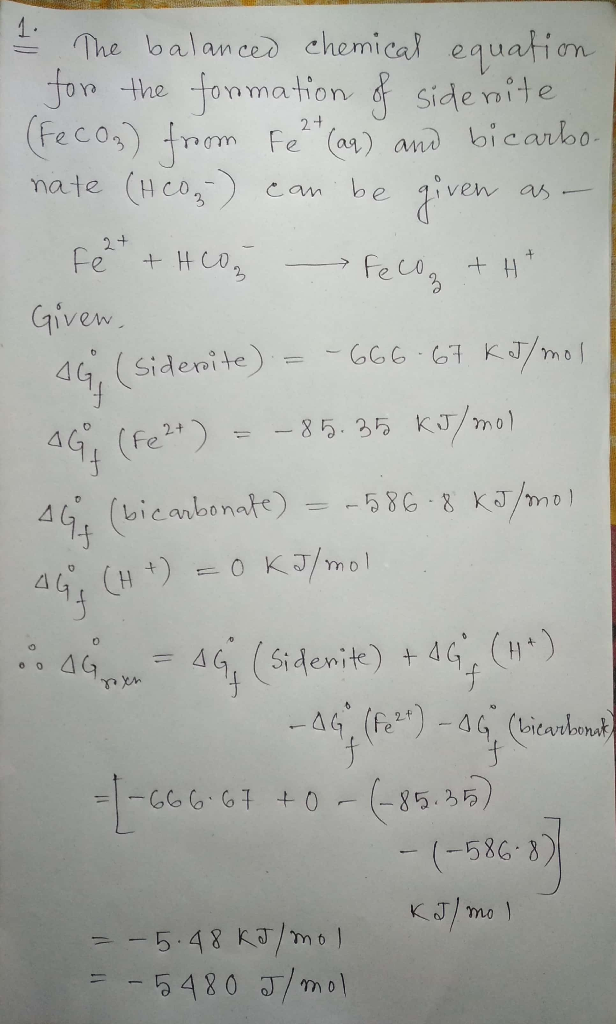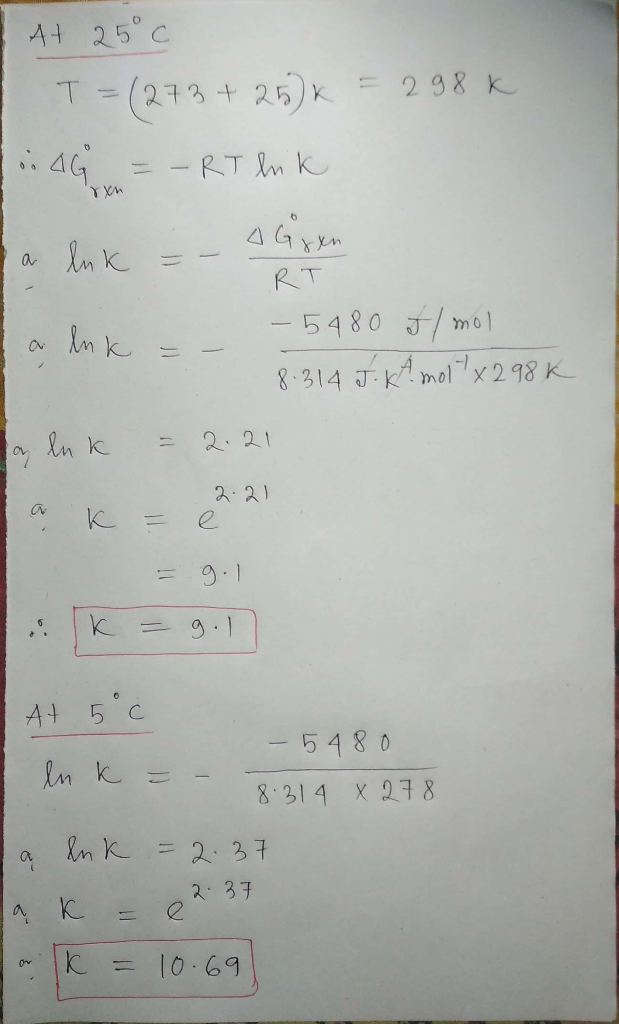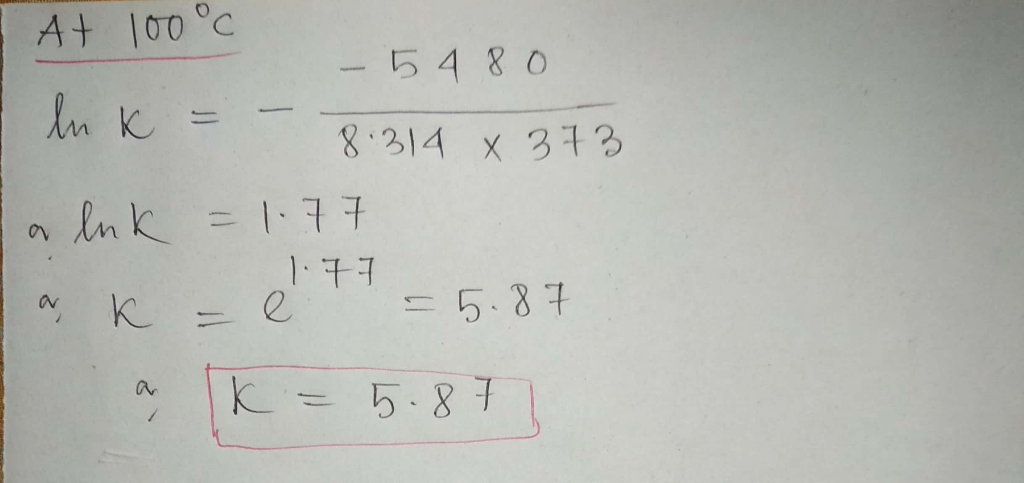##### Add Answer of: Useful Equations and Constants AG AG RTIn Q R 8.314olk F96485% vg > .G7,reactants 22, m Zi Ink互=...
Similar Homework Help Questions
• ### Useful Equations and Constants AG AG RTIn Q R 8.314olk F96485% vg > .G7,reactants 22, m Zi Ink互=...Useful Equations and Constants AG AG RTIn Q R 8.314olk F96485% vg > .G7,reactants 22, m Zi Ink互= Ea(T2-T1) 112 ΔΗ'(T2-T1) In- T1 =-T1T2 PROBLEM STATEMENT (100pts) With the following thermodynamic data, determine the K value at 25°C, 5°C, and 100°C for the formation of siderite (FeCOs) from Fe2 (aq) and bicarbonate (HCO;). To do so, first write the balanced equation. Next, find the ΔGorm, the ASorm, and the ΔΗ0rm. Finally give the K value for each temperature. 1. ΔG。--666.67...

• ### ΔΗ, (at 298K AG; (at 298 K in kJ/mol) Compound Compound AH (at 298K in kJ/mol in kJ/mol) AG (at 298 K. in kJ/mol...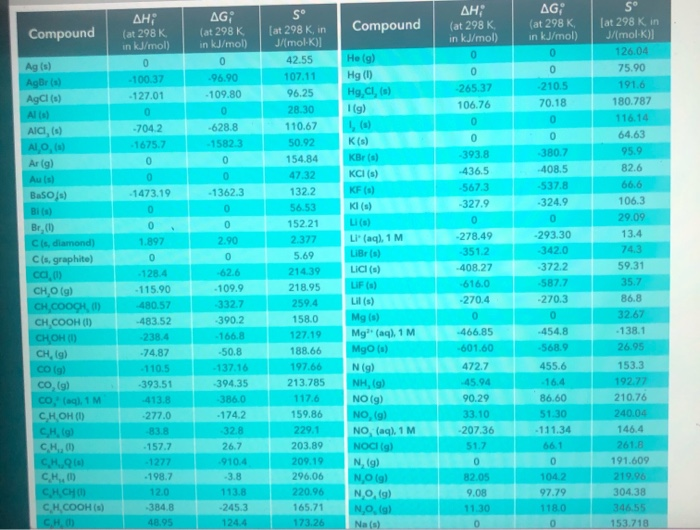ΔΗ, (at 298K AG; (at 298 K in kJ/mol) Compound Compound AH (at 298K in kJ/mol in kJ/mol) AG (at 298 K. in kJ/mol 0 0 210.5 70.18 Ho (g) Hg (1) Hg,CI, (s) Ag (s) Agor(s AgCl(s) 0 265.37 106.76 -96.90 -109.80 0 -628.8 - 1582.3 -100.37 -127.01 0 -704 2 -1675.7 0 1 (g) AICI, (s) Al O (5) Ar (9) Au (s) BaSO's) 0 K(s) KBr(s) KCI (s) 0 -393.8 436.5 -567.3 -327.9 0 -1362.3 380.7 -408.5...

Need Online Homework Help?